# Abel integral equation

(diff) ← Older revision | Latest revision (diff) | Newer revision → (diff)

The integral equation(1)

to which the solution of the Abel problem is reduced. The generalized Abel integral equation is the equation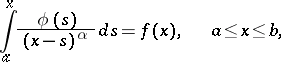(2)

where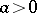and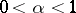are known constants,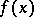is a known function andis the unknown function. The expressionis called the kernel of the Abel integral equation, or Abel kernel. An Abel integral equation belongs to the class of Volterra equations of the first kind (cf. Volterra equation). The equation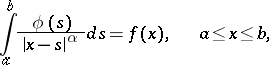(3)

is called Abel's integral equation with fixed limits.

Ifis a continuously-differentiable function, then the Abel integral equation (2) has a unique continuous solution given by the formula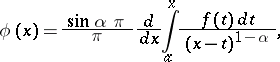(4)

or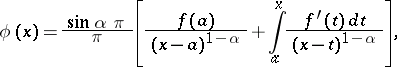(5)

which is the same thing. Formula (5) also gives the solution of an Abel integral equation (2) under more general assumptions , . Thus, it has been shown  that ifis absolutely continuous on the interval, the Abel integral equation (2) has the unique solution in the class of Lebesgue-integrable functions given by formula (5). For the solution of the Abel integral equation (3) see ; see also .

How to Cite This Entry:
Abel integral equation. Encyclopedia of Mathematics. URL: http://encyclopediaofmath.org/index.php?title=Abel_integral_equation&oldid=43531
This article was adapted from an original article by B.V. Khvedelidze (originator), which appeared in Encyclopedia of Mathematics - ISBN 1402006098. See original article